## Preview

Whole numbers are the numbers that you have been seeing all your life. All of math is connected with whole numbers, but most of all do you know why they are whole numbers?
These are whole numbers because they consist of no parts and are completely whole.
That also make the decimal point be at the end of the number.
So can you perform any mathematics with whole numbers? Of course you can!
Now try some of these and make sure to watch the video on YouTube.

Sheet 1 Logic behind Whole Numbers

## Preview

I bet you knew about from when you were in Kindergarten but did you ever come to realize that there are actually different types of triangles and each have a name.These are the triangles and their descriptions:

Obtuse Triangle-One angle is an obtuse angle.
These are all the six types of angles and the first three triangles are determined by the length of their sides and the other three are determined by the measurements of their angles.
So now you know that there are different types of triangles so the next time you spot one try and figure out which one it is.
Now try some of these and make sure to watch the video on YouTube.

## Example

#### What type of triangle is this?

1.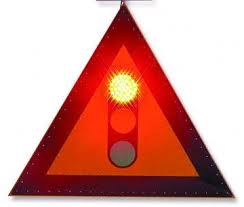=__________
2.=__________
3.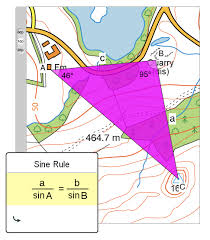=__________
4.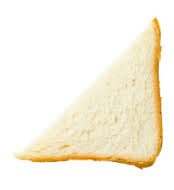=__________
5.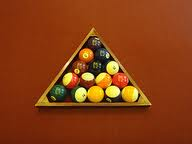=__________
6.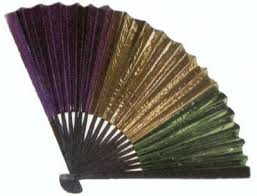=__________

Quadrilaterals are any shapes with 4 sides.
They can be classified by their angles, or by their pairs of sides.

• Parallelogram
• Trapezoid
• Rectangle
• Rhombus
• Square

• A trapezoid has exactly one pair of parallel sides.
• A parallelogram has two pairs of opposite sides: parallel and equal
• A rectangle has four right angles
• A rhombus has all sides the same length
• A square has all sides the same length and has four right angles
Sheet 1 What are the Properties of Quadrilaterals

Sheet 2 What are the Properties of Quadrilaterals

## Polygons

A polygon is a closed figure, or shape, made up of line segments.

There are two types of polygon classifications:
Regular Polygons: sides of equal length and angles of equal measurements
Irregular Polygons: sides of different length and angles of different measurements

Sheet 1 What are Polygons

Sheet 2 What are Polygons

## Preview

Plane shapes are the shapes that you probably know from Kindergarten, they are the squares, triangles, rectangles etc.
But what is so special about these shapes. Well these shapes are shapes that are 2-dimensional shapes. They have a length and width but they do not have a height. So now you know the importance of plane shapes.
Now try some of these and make sure to watch the video on YouTube.

Sheet 1 Learn about Plane Shapes

Sheet 2 Learn about Plane Shapes

## Preview

Graphs are the best way to present data in a organized way.
There are many types of graphs and all of them serve a different purpose.
The different graphs are used by the type of information given.
So for example if you have a voting in a class and the information is given to graph then the best types of graphs to use would be line plots, bar graphs,  and circle graphs.
Now review this information and make sure to watch the video on YouTube.

## Example

1.Bar Graph## "Graph" Decisions

Graphs are a great source to display information, but they also help us in making decisions on that topic. For example, let's say that a pie graph represents the different methods of transportation.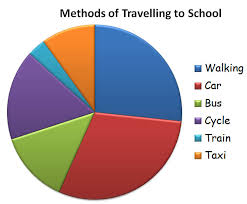This will help us in making decisions like:
• Most students walk to school
• Only a few students take the train to school
• The same amount of students take the car to school as the amount of students who walk to school.

## Preview

Line plots are a quick way to organize things and they are also easy to make. They look something like this:

Line plots can be used to show organization in many situations. For example if you want to show how many students like a color then you can write the color names on the bottom of the line and the number of students who like each color can be represented as x's.
Now try some of these and make sure to watch the video on YouTube.

2.

• Line Graphs
• Bar Graphs
• Pictographs
• Pie Charts

## What is the Main Method for Collecting Data?

The main method for collecting data is through surveys.

A survey is a question, or many questions, used to gather information called data.

## Data from Surveys

Mr. Carter's students got the following scores on their math test:

67, 98, 77, 87, 83, 91, 55, 70, 95, 75

To display this data, we can use many different methods, some of which include making line plots.
Line plots allow you to display your data in an orderly fashion.

## Line Plots: Examples

Here are some example line plots with collected data from different samples:

## Introduction

Conversion between metric units and customary units is really easy...as long as you use this chart:
This is the basic conversion between the two units of measures. This chart can help you to convert any other measure!

## Convert Metric to Customary

Let's say that a baby weighs 30 pounds.
How many grams does the baby weigh?
The first thing you do is look at the chart: we already know that one pound equals 454 grams. All we do now is just simply multiply 454x30=13,620 grams.
That means that the baby weighs 13,620 grams!

Sheet 1 Learn the Conversion between Metric Units and Customary Units

## Preview

To start this introduction you need to know there are three types of metric units: Metric Units of Length,
Metric Unit of Capacity, and Metric Units of Mass. The first is units of length and that includes of:

Next is the units of capacity which includes of:Third is the units of mass which include of: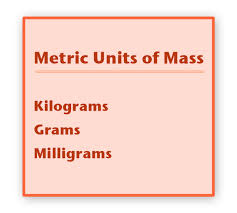All of these units are uses of everyday life so knowing them really gives you a benefit of calculating things more easily.
For example you will use meters from the units of lengths to find out how much you walked.
You will use cups from the units of capacity to calculate how much you need to put in your recipe.
You will use kilograms from the units of mass to find out how much weight you have lost.
Now try some of these and make sure to watch the video on YouTube.

Sheet 1 What are Metric Units